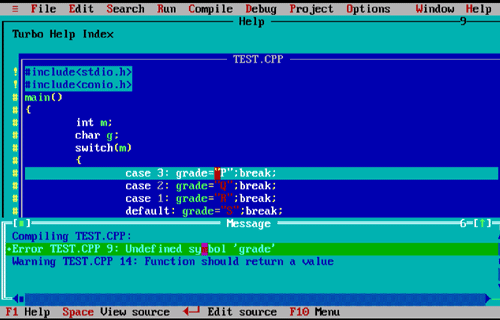# MCQs on Conditional Statements & Loops - C Programming Multiple Choice questions with answers

Questions
1 Assume that i,j and k are integer variables and their values are 8, 5 and 0 respectively. What will be the values of variables i and k after executing the following expression?

```k=(j>5)?(i<5)?i-j:j-i:k-j;
i-=(k)?(i)?(j)?:(i):(k);
```

What will be the result of execution?

A -3 and 3
B 3 and -5
C 3 and -3
D -5 and 3

Given that the values of i,j and k are 8, 5 and 0 respectively. The first statement j>5 is not executed because j=5 and i<5 is also not executed. So the program evaluate k-j, which is, 0-5=-5. So afetre execution of first expression the values are 8, 5 and -5 respectively.

In the second statement the values of k, i are non zero. so it is true for both. So the values of i, j and k are 3, 5 and -5

2 Find the error in the following program:

```main()
{
int m;
char g;
switch(m)
{
}
}
```

What will be the output of the program?

B switch statement cannot have more than three labels
C case label cannot be numbers
D none of these3 Data type of the controlling statement of a SWITCH statement cannot of the type:
A int
B char
C short
D float

The SWITCH statement is a conditional statement. Using this statement we handle number of cases simultaneously. Float data type is not allowed in SWITCH statement.

4 How long the following loop runs?

```for(x=0;x=3;x++)
```
A Three times
B Four times
C Forever
D Never

The first statement of a for loop is initialize the loop counter, second is conditional statment and the third one is increment/decrement of the loop counter.

In the given expression the second statement is an assignment statement instead of condition.

So the for loop never execute.

5 The CONTINUE statemment cannot be used with
A for
B switch
C do
D while

The CONTINUE Keyword skips the code and immediately passes control to the beginning of the statement for next iteration.

### Chapters